In force

Version from: 01/01/2023
Amendments
Search within this legal act

### Article 280a - Interest rate risk category add-on

Article 280a

1.

For the purposes of Article 278, institutions shall calculate the interest rate risk category add-on for a given netting set as follows: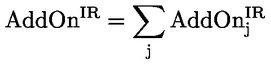where:

 j = the index that denotes all the interest rate risk hedging sets established in accordance with point (a) of Article 277a(1) and with Article 277a(2) for the netting set; and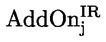= the interest rate risk category add-on for hedging set j calculated in accordance with paragraph 2.
2.

Institutions shall calculate the interest rate risk category add-on for hedging set j as follows: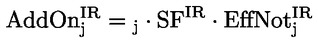where:

 єj = the hedging set supervisory factor coefficient of hedging set j determined in accordance with the applicable value specified in Article 280;
 SFIR = the supervisory factor for the interest rate risk category with a value equal to 0,5 %; and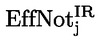= the effective notional amount of hedging set j calculated in accordance with paragraph 3.
3.

For the purpose of calculating the effective notional amount of hedging set j, institutions shall first map each transaction of the hedging set to the appropriate bucket in Table 2. They shall do so on the basis of the end date of each transaction as determined under point (a) of Article 279b(1):

Table 2

 Bucket End date (in years) 1 > 0 and <= 1 2 > 1 and <= 5 3 > 5

Institutions shall then calculate the effective notional amount of hedging set j in accordance with the following formula: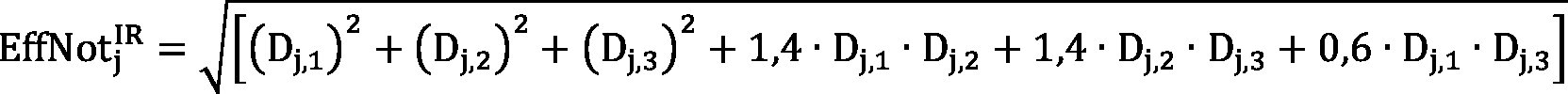where:= the effective notional amount of hedging set j; and
 Dj,k = the effective notional amount of bucket k of hedging set j calculated as follows: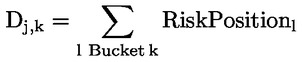where:

 l = the index that denotes the risk position.